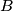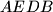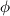### IMO Shortlist 2013 problem G3

Kvaliteta:
Avg: 0,0
Težina:
Avg: 7,0
In a triangle$ABC$, let$D$ and$E$ be the feet of the angle bisectors of angles$A$ and$B$, respectively. A rhombus is inscribed into the quadrilateral$AEDB$ (all vertices of the rhombus lie on different sides of$AEDB$). Let$\phi$ be the non-obtuse angle of the rhombus. Prove that$\phi \leq \operatorname{max}\{ \angle BAC, \angle ABC \}$.
Izvor: Serbia